output.to from Sideway
Draft for Information Only

# Content

```Instantaneous Rate of Change  Circular Motion     Rotational and Translational Motion   Circular Motion Problem```

# Instantaneous Rate of Change

In linear motion, the instantaneous rate of change is related to the physical displacement of an object with respect to time. But the instantaneous rate of change can also be related to the travelled angular displacement or tangential distance of an rotating object with respect to the angle of rotation in radians or with respect to time.

## Circular Motion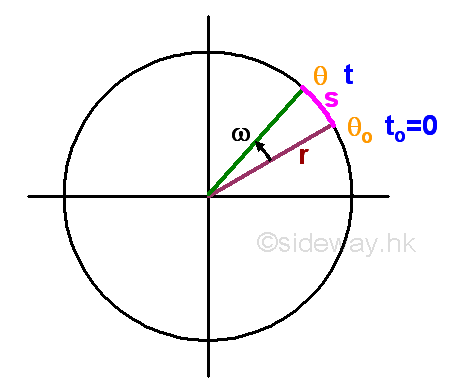For a uniform circular motion, the object is moving in a circular path. When the object rotates from one position to another about the centre of rotation with radius r, the angle c measured in radians formed between the initial and final position of the object with the centre of rotation that the rotating object experiences is called the angular displacement or angular distance.

For a circular motion with uniform angular speed ω, the relationship between the travelled angular distance θ, initial angular distance θo, angular speed ω and time t can be expressed as a function: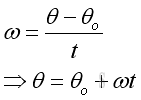Instantaneous angular velocity can be defined as the instantaneous rate of change of the travelled angular distance with respect to the time of travelling. The derivative of the function θ with respect to time t is.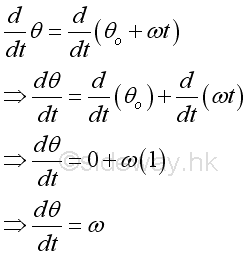For a circular motion with constant angular acceleration α, the relationship between the final angular speed ω, initial angular speed ωo, angular acceleration α and time t can be expressed as a function: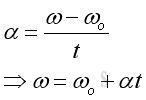Instantaneous angular acceleration can be defined as the instantaneous rate of change of the travelling velocity with respect to the time of travelling. The derivative of the function ω with respect to time t is.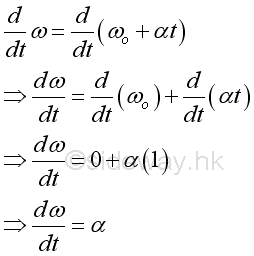Since θ is a function of time f(t), the derivative ω is equal to the first derivative of function θ with respect to time t.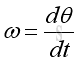The derivative α is equal to the derivative of function ω with respect to time t. But ω is also a derivative of function θ with respect to time t, the derivative is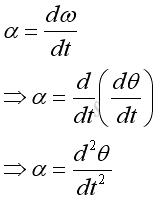Therefore the derivative α is also equal to the second derivative of function θ with respect to time t.

### Rotational and Translational Motion

And the travelled distance s or the angular distance is equal to the length of the angular path through which it moves along with.  The relationship between the travelled distance s, radius r and angular displacement θ can be expressed as a function: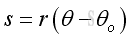Since both the angular distance s and angular displacement θ are functions of time t, the instantaneous rate of change of the angular distance s with respect to time are also a function of the instantaneous rate of change of the angle θ of the angular displacement measured in radians. And they are related by the radius r of the circular motion. The derivative of the function s with respect to time t is.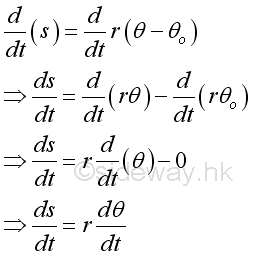Therefore the instantaneous linear velocity is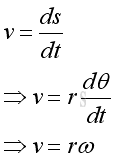Since s is a function of time f(t), the derivative v is equal to the first derivative of function s with respect to t.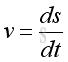The derivative a is equal to the derivative of function v with respect to time t. But v is also a derivative of function s with respect to time t, the derivative is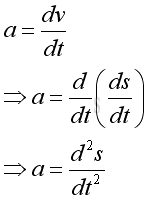Therefore the derivative a is also equal to the second derivative of function s with respect to time t. Besides, the instantaneous linear acceleration can also be expressed in terms of angular displacement, imply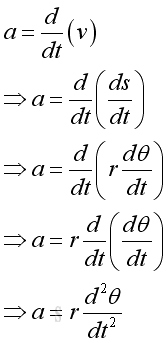### Circular Motion Problem

For an object with a circular motion and the motion can be described by a function θ with respect to time t.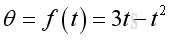Graphically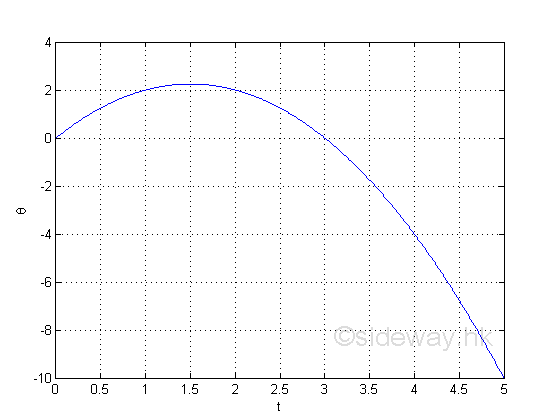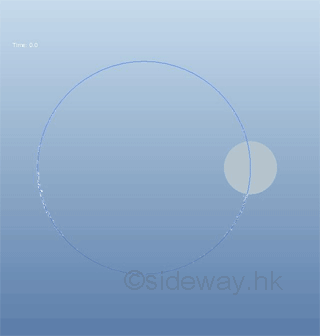The information directly from the function is the travelled angular distance from the original position.

e.g. at t=1, the angular distance from the original position is 2,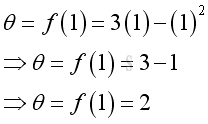e.g. at t=2, the angular distance from the original position is 2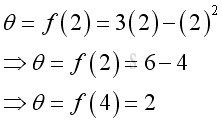e.g. at t=4, the angular distance from the original position is -4, the negative sign means the angular distance is measured from the opposite direction of the original position relative to the original default measurement.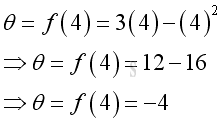The first derivative can be used to determine the instantaneous angular verlocity of the object motion. imply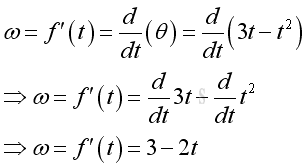Graphically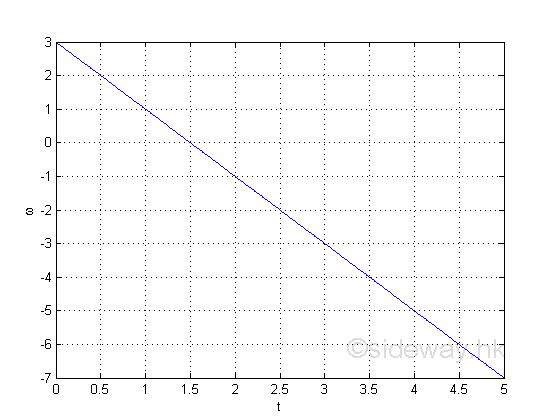The information directly from the derivative is the instantaneous angular velocity.

e.g. at t=1, the instantaneous angular velocity is 1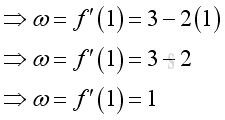e.g. at t=2, the instantaneous velocity is -1, the negative sign means the direction of motion is opposite to the originial direction of the default motion.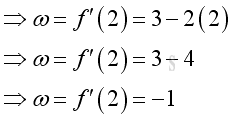e.g. In stationary state condition, the instantaneous angular velocity of the object is eqaul to zero. Imply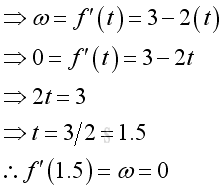Therefore at t=1.5, the object remains stationary at its position with a zero instantaneous angular velocity. Imply the position is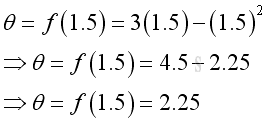The second derivative can be used to determine the instantaneous angular acceleration of the object motion. imply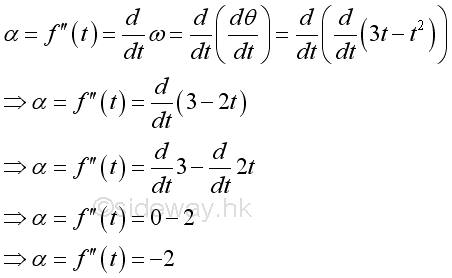The angular accelation is equal to -2, a negative angular acceleration. The value is a negative constant which imply it is a constant deceleration. And therefore the instantaneous angular velocity is decreasing with respect to time.  Graphically, the angular acceleration is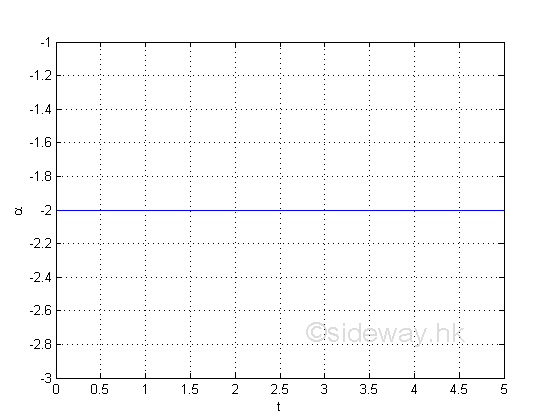ID: 110900015 Last Updated: 22/9/2001 Revision: 0 Ref:References

1. S. James, 1999
2. B. Joseph, 1978Home 5

Management

HBR 3

Information

Recreation

Culture

Chinese 1097

English 337

Computer

Hardware 149

Software

Application 187

Numeric 19

Programming

Web 757

CSS 1

HTML

Knowledge Base

OS 389

MS Windows

Knowledge

Mathematics

Algebra 20

Geometry 18

Calculus 67

Engineering

Mechanical

Rigid Bodies

Statics 92

Dynamics 37

Control

Physics

Electric 10